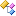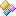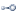﻿ Accord.Statistics.Distributions.Multivariate Namespace# Accord.Statistics.Distributions.Multivariate Namespace

Contains a multivariate distributions such as the multivariate Normal, Multinomial, Independent, Joint and Mixture distributions.Classes
ClassDescriptionDirichletDistribution
Dirichlet distribution.HiddenMarkovDistribution
Hidden Markov Model probability distribution.Independent
Joint distribution assuming independence between vector components.IndependentTDistribution
Joint distribution assuming independence between vector components.IndependentTDistribution, TObservation
Joint distribution assuming independence between vector components.IndependentTDistribution, TObservation, TOptions
Joint distribution assuming independence between vector components.InverseWishartDistribution
Inverse Wishart Distribution.JointDistribution
Joint distribution of multiple discrete univariate distributions.MatrixContinuousDistribution
Abstract class for Matrix Probability Distributions.MultinomialDistribution
Multinomial probability distribution.MultivariateContinuousDistribution
Abstract class for Multivariate Probability Distributions.MultivariateDiscreteDistribution
Abstract class for multivariate discrete probability distributions.MultivariateEmpiricalDistribution
Multivariate empirical distribution.MultivariateMixtureT
Mixture of multivariate probability distributions.MultivariateNormalDistribution
Multivariate Normal (Gaussian) distribution.UniformBallDistribution
Uniform distribution inside a n-dimensional ball.UniformSphereDistribution
Uniform distribution inside a n-dimensional ball.VonMisesFisherDistribution
Von-Mises Fisher distribution.WishartDistribution
Wishart Distribution.Structures
StructureDescriptionMixtureComponentT
Represents one component distribution in a mixture distribution.Interfaces
InterfaceDescriptionIMixtureComponentTRemarks

The namespace class diagram is shown below.See Also